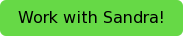On the AP Bio exam, there will be 6 grid-in questions that will require you to do some math. Yes, math. These questions will require you to do some simple calculations and thus you are only allowed a four-function calculator to do addition, subtraction, division, and multiplication. That being said I’ll breakdown an example grid-in question to familiarize students with the type of questions they may be asked.

In corn (Zea mays), yellow kernels (Y) are dominant to red kernels (y). A plant with yellow kernels was crossed to a plant with red kernels and the resulting cobs were analyzed in the lab. A student counts 329 yellow and 299 red kernels on one cob.

Calculate the chi-squared value for the null hypothesis that the yellow parent was heterozygous for yellow kernels. Give your answer to the nearest tenth.Let’s review the chi-squared test. This statistical test is used to quantify the deviations expected by chance if a hypothesis is true. So in simple terms the analysis allows you determine whether something is happening by chance or whether the differences that are seen are due to more than just chance. In this case, we are trying to quantify whether or not the offspring of a cross between two parents fits a Mendelian distribution. The null hypothesis in this question is that the yellow parent is heterozygous for the dominant yellow kernels (Yy). If we accept this null hypothesis, it means that any deviations we see are just due to chance. If we reject the null hypothesis, it means that are results are probably not due to chance and that some real difference is occurring.

The first thing we need to do is figure out the numbers expected from a cross between a heterozygous yellow parent (Yy) and the recessive red kernel plant (yy).With this cross we expect to get a 1:1 ration between yellow kernels and red kernels. So, when the total number of kernels counted is 628 (329+299), we expect that 314 of these kernels will be yellow and 314 will be red. What actually is observed is that 329 kernels are yellow and 299 kernels are red. To perform the chi-square test to determine whether the differences we observed are just due to chance will we also need the degrees of freedom. The degrees of freedom is just the number of categories minus 1. In our case, that would be two (yellow or red) minus 1, which is 1 degree of freedom. The equation for chi-squared is given on the equation sheet that is provided. It is:O being observed and e being the expected values. If we plug our data into this equation we have Χ2 = Σ(329-314)2/314 + (299-314)2/314. Χ2= (0.72) + (0.72) = 1.44. Using the chi-squared degrees of freedom table that is also given during the exam we see that for 1 degree of freedom a p value of 0.05 needs to be greatet than 3.84 to accept the null hypothesis and less than 3.84 to reject. Since our p value is 1.44 which is less than 3.84, we can reject the null hypothesis and conclude that a factor besides chance is contributing to the differences in the expected versus observed colors of the kernels. For the grid however you would just put in the chi-squared value that you calculated as 1.44

This question is adapted from www.clearbiology.com where there are many resources related to the AP Bio exam.

Are you interested in working with Sandra on preparing for the AP Biology exam?Read more tips on how to approach the AP Biology exam below!

## How to Study for AP Biology – a General Method

Tags: biology, AP exams